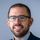cancel
Showing results for
Did you mean:Frequent Visitor

## Average \$ per weekday

I have a table of cash receipts with a date column (i.e., 1/1/2022...4/4/2022). I'd like to calculate the average amount received by week day. So if there were 3 Mondays in the date range and the total collected on those Mondays was \$3,000,000, I'd expect to see an output like this:

Weekday | Average

---------------------

Mon           \$1,000,000

1 ACCEPTED SOLUTIONSuper User

Hi @mcmullenrich
Sorry for the late reply. Here is the workable solution as per sample sample

``````Average of Amt :=
DIVIDE (
SUM ( Combined[Amount] ),
COUNTROWS ( FILTER ( 'Calendar', NOT ISBLANK ( Combined[Sum of Amt] ) ) )
)``````
15 REPLIES 15Super User

you can create a new calculated column for weekday

FORMAT ( WEEKDAY(Table[Date]), "dddd" )

then use it in your visual with simple AVERAGE MeasureFrequent Visitor

Thanks @tamerj1. I tried this and got the average of all the individual transaction instead of the average daily total for each day.Super User

Try

DIVIDE (SUM (Table[Value]), CALCULATE (SUM(Table[Value]), ALLSELECTED(Table[Weekday])))Frequent Visitor

Hmm...that got me a 1 for each day. I think I'm using the wrong Table[Value] on one of them:

Average per Weekday :=
DIVIDE (
SUM ( Combined[Amount] ),
CALCULATE ( SUM ( Combined[Amount] )ALLSELECTED ( 'Calendar'[Day Of Week] ) ),
0
)Super User

I believe I misunderstood your reqyirement. You just want to divide over the number of frequency of the selected weeday. Therefore if you are using a date table just do:

Average per Weekday :=

DIVIDE (
SUM ( Combined[Amount] ),
COUNTROWS ( 'Calendar' )
)Frequent Visitor

I'm going to try this when I get home. That said, to ensure I understand it and you understand what I'm looking to do, here is how I would solve it in excel:

Add a column called "Weekday" with formula =WEEKDAY(Date)

Off to the right, create a small table with numbers 1 through 7 as rows

Create columns Total (=SUMIF(Weekday, Day, Amount) and Count (=COUNTIF(Weekday, Day)

Divide Total by Count

This would give me the total deposits on each weekday and divide it by the number of occurrences of each workday for an average daily total for each weekday.Super User

That is clear enough. But in Power Bi you have a date table with the weekday name column and this table is connected with the fact table through the date column. Is that correct?Frequent Visitor

Yes, that's correctSuper User

Then I hope the last solution works.Frequent Visitor

Not quite there yet. I realized the issue, just not sure how to solve in DAX. My excel-based approach above only works if I have a single entry for each date (i.e., a daily deposit amount). However, in my PBI table, I have multiple transactions for each date. As such, your approach gives me much smaller numbers than it should be. I need to find a way to sum by date before adding and counting.Super User

@mcmullenrich
Even though, COUNTROWS ( 'Calendar' ) should give the frequency count of the selected weekday as the date table has no duplicates. Can you please share some screenshots of you tabels, data model, report and the measure?Frequent Visitor

This feels a bit like I cheated, but it did get me the result that I wanted:

Average per Weekday :=
DIVIDE ( [Sum of Amt], MAX ( Combined[WeekNo] ) - MIN ( Combined[WeekNo] ) + 1 )Super User

Hi @mcmullenrich
Sorry for the late reply. Here is the workable solution as per sample sample

``````Average of Amt :=
DIVIDE (
SUM ( Combined[Amount] ),
COUNTROWS ( FILTER ( 'Calendar', NOT ISBLANK ( Combined[Sum of Amt] ) ) )
)``````Super User

I'd suggest to create a date dimension in Power BI where one of the attributes is weekday. Here you have a blog post where they explain how to create such a date dimension.
Power Bi for Beginners: How to create a Date Table in Power Bi - Softcrylic

Afterwards, create an Average measure and drag in the WeekDay attribute into your visual.

Let me know if this helps or if yiou have any other questions 🙂

Proud to be a Super User!Frequent Visitor

Thanks @tackytechtom. So I'm actually working in PowerPivot, not PowerBI. I figured the DAX would be the same, but maybe not. I created a date table and have established the relationship between my transaction table and it. However, the closest I have come to getting the right output is to get the total deposits by weekday divided by the 7 days in the week instead of the 14 distinct occurences of each weekday.